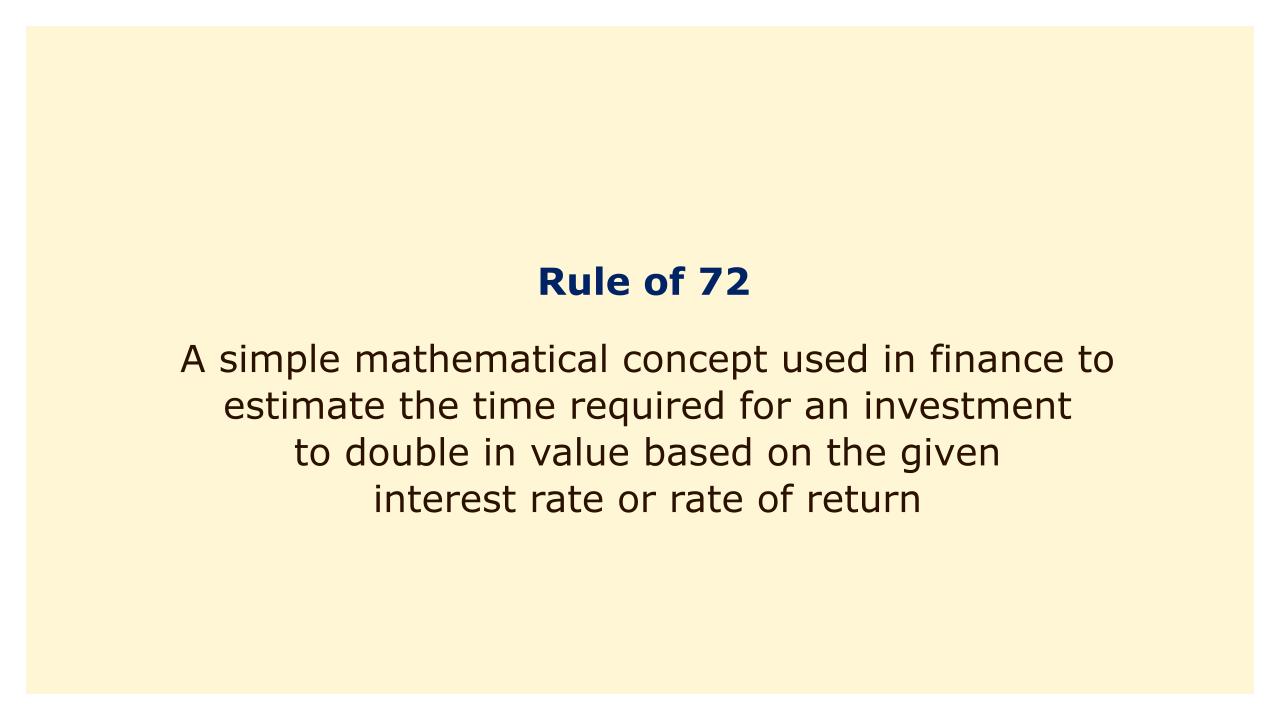# Rule of 72Image: Moneybestpal.com

### A simple mathematical concept known as the Rule of 72 is used in finance to calculate how long it will take for an investment to double in value given the current interest rate or rate of return. The rule indicates that 72 divided by the yearly rate of return is roughly equivalent to the number of years it takes for an investment to double in value.

If an investment, for instance, had an annual rate of return of 6%, it would take about 12 years for the investment's value to double, which is determined by multiplying 72 by 6%. Similarly to this, if an investment yields 9% annually, it would take around 8 years for its value to double, which is determined by dividing 72 by 9.

Investors frequently apply this method to analyze various investment possibilities and gauge the prospective development of their capital. It is crucial to keep in mind that the rule is simply a rough estimate and does not account for elements like inflation, taxes, and other expenses that could alter the real return on investment.
Tags